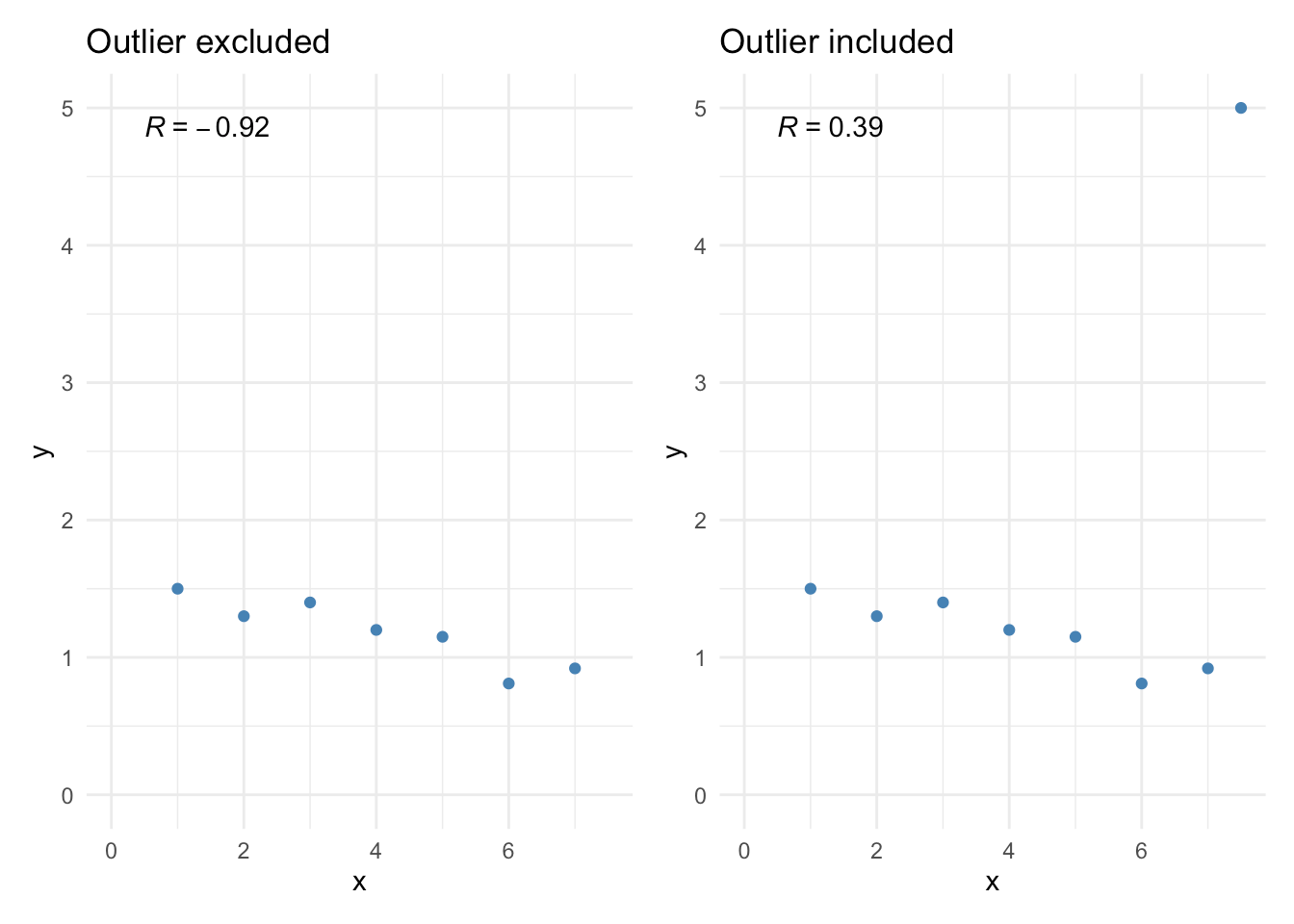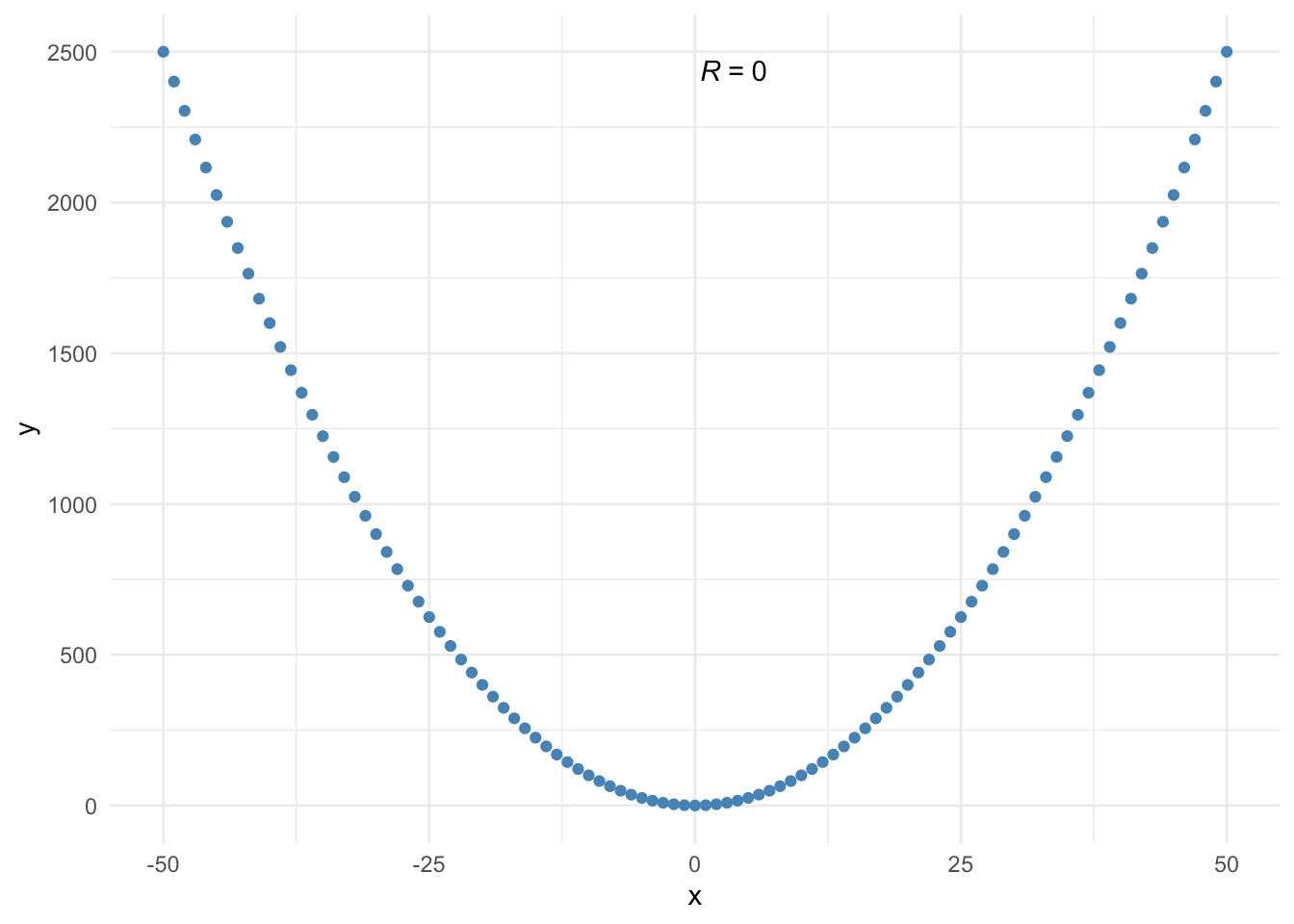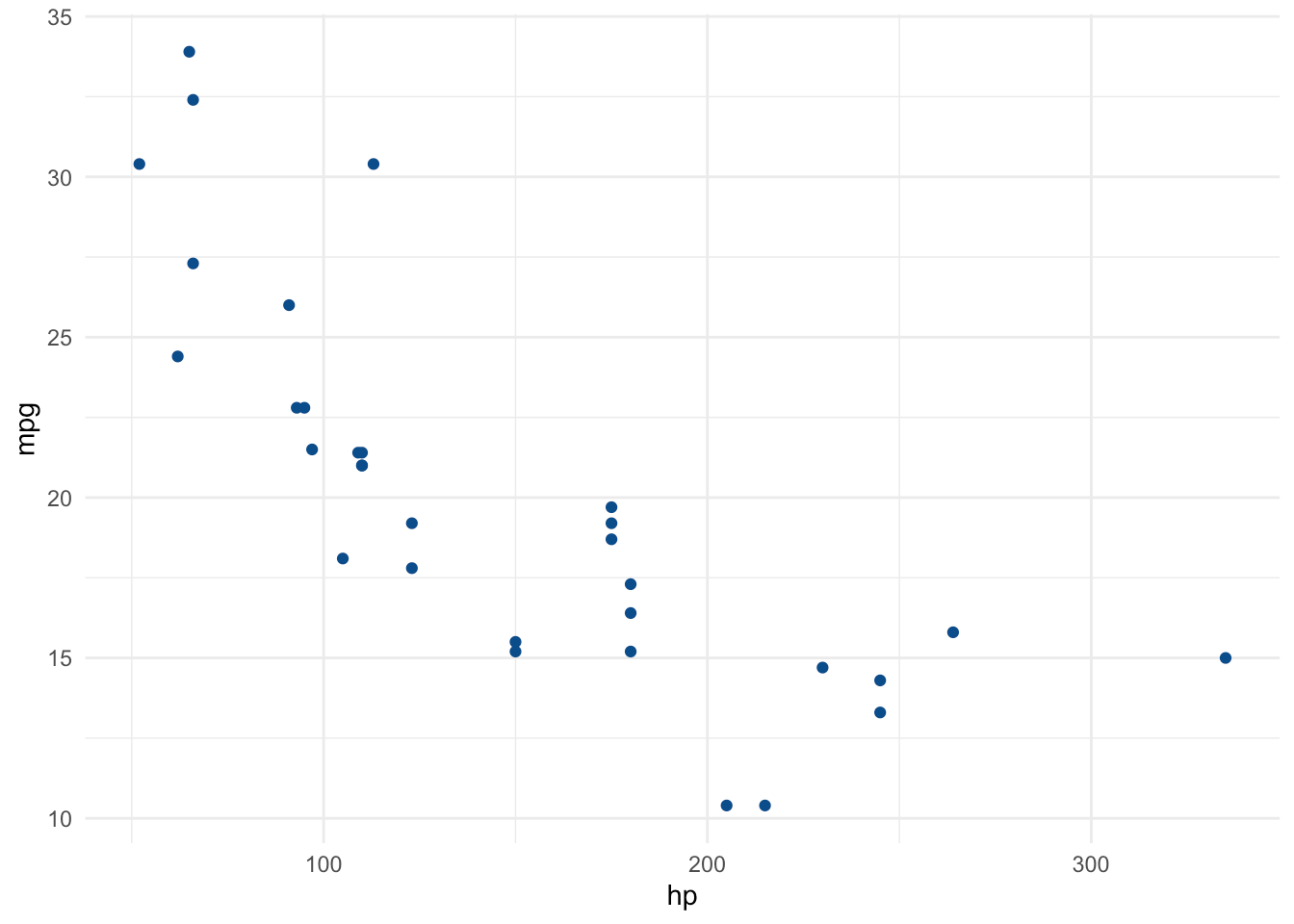Want to share your content on R-bloggers? click here if you have a blog, or here if you don't.# Introduction

Correlations between variables play an important role in a descriptive analysis. A correlation measures the relationship between two variables, that is, how they are linked to each other. In this sense, a correlation allows to know which variables evolve in the same direction, which ones evolve in the opposite direction, and which ones are independent.

In this article, I show how to compute correlation coefficients, how to perform correlation tests and how to visualize relationships between variables in R.

Correlation is usually computed on two quantitative variables. See the Chi-square test of independence if you need to study the relationship between two qualitative variables.

# Data

In this article, we use the mtcars dataset (loaded by default in R):

# display first 5 observations
head(mtcars, 5)
##                    mpg cyl disp  hp drat    wt  qsec vs am gear carb
## Mazda RX4         21.0   6  160 110 3.90 2.620 16.46  0  1    4    4
## Mazda RX4 Wag     21.0   6  160 110 3.90 2.875 17.02  0  1    4    4
## Datsun 710        22.8   4  108  93 3.85 2.320 18.61  1  1    4    1
## Hornet 4 Drive    21.4   6  258 110 3.08 3.215 19.44  1  0    3    1
## Hornet Sportabout 18.7   8  360 175 3.15 3.440 17.02  0  0    3    2

The variables vs and am are categorical variables, so they are removed for this article:

# remove vs and am variables
library(tidyverse)
dat <- mtcars %>%
select(-vs, -am)

# display 5 first obs. of new dataset
head(dat, 5)
##                    mpg cyl disp  hp drat    wt  qsec gear carb
## Mazda RX4         21.0   6  160 110 3.90 2.620 16.46    4    4
## Mazda RX4 Wag     21.0   6  160 110 3.90 2.875 17.02    4    4
## Datsun 710        22.8   4  108  93 3.85 2.320 18.61    4    1
## Hornet 4 Drive    21.4   6  258 110 3.08 3.215 19.44    3    1
## Hornet Sportabout 18.7   8  360 175 3.15 3.440 17.02    3    2

# Correlation coefficient

## Between two variables

The correlation between 2 variables is found with the cor() function. Suppose we want to compute the correlation between horsepower (hp) and miles per gallon (mpg):

# Pearson correlation between 2 variables
cor(dat$hp, dat$mpg)
##  -0.7761684

Note that the correlation between variables x and y is equal to the correlation between variables y and x so the order of the variables in the cor() function does not matter.

The Pearson correlation is computed by default with the cor() function. If you want to compute the Spearman correlation, add the argument method = "spearman" to the cor() function:

# Spearman correlation between 2 variables
cor(dat$hp, dat$mpg,
method = "spearman"
)
##  -0.8946646

While Pearson correlation is often used for quantitative continuous variables, Spearman correlation (which is based on the ranked values for each variable rather than on the raw data) is often used to evaluate relationships involving ordinal variables. Run ?cor for more information about the different methods available in the cor() function.

## Correlation matrix: correlations for all variables

Suppose now that we want to compute correlations for several pairs of variables. We can easily do so for all possible pairs of variables in the dataset, again with the cor() function:

# correlation for all variables
round(cor(dat),
digits = 2 # rounded to 2 decimals
)
##        mpg   cyl  disp    hp  drat    wt  qsec  gear  carb
## mpg   1.00 -0.85 -0.85 -0.78  0.68 -0.87  0.42  0.48 -0.55
## cyl  -0.85  1.00  0.90  0.83 -0.70  0.78 -0.59 -0.49  0.53
## disp -0.85  0.90  1.00  0.79 -0.71  0.89 -0.43 -0.56  0.39
## hp   -0.78  0.83  0.79  1.00 -0.45  0.66 -0.71 -0.13  0.75
## drat  0.68 -0.70 -0.71 -0.45  1.00 -0.71  0.09  0.70 -0.09
## wt   -0.87  0.78  0.89  0.66 -0.71  1.00 -0.17 -0.58  0.43
## qsec  0.42 -0.59 -0.43 -0.71  0.09 -0.17  1.00 -0.21 -0.66
## gear  0.48 -0.49 -0.56 -0.13  0.70 -0.58 -0.21  1.00  0.27
## carb -0.55  0.53  0.39  0.75 -0.09  0.43 -0.66  0.27  1.00

This correlation matrix gives an overview of the correlations for all combinations of two variables.

## Interpretation of a correlation coefficient

First of all, correlation ranges from -1 to 1.

On the one hand, a negative correlation implies that the two variables under consideration vary in opposite directions, that is, if a variable increases the other decreases and vice versa. On the other hand, a positive correlation implies that the two variables under consideration vary in the same direction, i.e., if a variable increases the other one increases and if one decreases the other one decreases as well. Last but not least, a correlation close to 0 indicates that the two variables are independent.

As an illustration, the Pearson correlation between horsepower (hp) and miles per gallon (mpg) found above is -0.78, meaning that the 2 variables vary in opposite direction. This makes sense, cars with more horsepower tend to consume more fuel (and thus have a lower millage par gallon). On the contrary, from the correlation matrix we see that the correlation between miles per gallon (mpg) and the time to drive 1/4 of a mile (qsec) is 0.42, meaning that fast cars (low qsec) tend to have a worse millage per gallon (low mpg). This again make sense as fast cars tend to consume more fuel.

The correlation matrix is however not easily interpretable, especially when the dataset is composed of many variables. In the following sections, we present some alternatives to the correlation matrix.

# Visualizations

## A scatterplot for 2 variables

A good way to visualize a correlation between 2 variables is to draw a scatterplot of the two variables of interest. Suppose we want to examine the relationship between horsepower (hp) and miles per gallon (mpg):

# scatterplot
library(ggplot2)

ggplot(dat) +
aes(x = hp, y = mpg) +
geom_point(colour = "#0c4c8a") +
theme_minimal()If you are unfamiliar with the {ggplot2} package, you can draw the scatterplot using the plot() function from R base graphics:

plot(dat$hp, dat$mpg)or use the esquisse addin to easily draw plots using the {ggplot2} package.

## Scatterplots for several pairs of variables

Suppose that instead of visualizing the relationship between only 2 variables, we want to visualize the relationship for several pairs of variables. This is possible thanks to the pair() function. For this illustration, we focus only on miles per gallon (mpg), horsepower (hp) and weight (wt):

# multiple scatterplots
pairs(dat[, c(1, 4, 6)])The figure indicates that weight (wt) and horsepower (hp) are positively correlated, whereas miles per gallon (mpg) seems to be negatively correlated with horsepower (hp) and weight (wt).

## Another simple correlation matrix

This version of the correlation matrix presents the correlation coefficients in a slightly more readable way, i.e., by coloring the coefficients based on their sign. Applied to our dataset, we have:

# improved correlation matrix
library(corrplot)

corrplot(cor(dat),
method = "number",
type = "upper" # show only upper side
)# Correlation test

## For 2 variables

Unlike a correlation matrix which indicates correlation coefficients between pairs of variables, the correlation test is used to test whether the correlation (denoted $$\rho$$) between 2 variables is significantly different from 0 or not.

Actually, a correlation coefficient different from 0 does not mean that the correlation is significantly different from 0. This needs to be tested with a correlation test. The null and alternative hypothesis for the correlation test are as follows:

• $$H_0$$: $$\rho = 0$$
• $$H_1$$: $$\rho \ne 0$$

Suppose that we want to test whether the rear axle ratio (drat) is correlated with the time to drive a quarter of a mile (qsec):

# Pearson correlation test
test <- cor.test(dat$drat, dat$qsec)
test
##
##  Pearson's product-moment correlation
##
## data:  dat$drat and dat$qsec
## t = 0.50164, df = 30, p-value = 0.6196
## alternative hypothesis: true correlation is not equal to 0
## 95 percent confidence interval:
##  -0.265947  0.426340
## sample estimates:
##        cor
## 0.09120476

The p-value of the correlation test between these 2 variables is 0.62. At the 5% significance level, we do not reject the null hypothesis of no correlation. We therefore conclude that we do not reject the hypothesis that there is no linear relationship between the 2 variables.

This test proves that even if the correlation coefficient is different from 0 (the correlation is 0.09), it is actually not significantly different from 0.

Note that the p-value of a correlation test is based on the correlation coefficient and the sample size. The larger the sample size and the more extreme the correlation (closer to -1 or 1), the more likely the null hypothesis of no correlation will be rejected. With a small sample size, it is thus possible to obtain a relatively large correlation (based on the correlation coefficient), but still find a correlation not significantly different from 0 (based on the correlation test). For this reason, it is recommended to always perform a correlation test before interpreting a correlation coefficient to avoid flawed conclusions.

## For several pairs of variables

Similar to the correlation matrix used to compute correlation for several pairs of variables, the rcorr() function (from the {Hmisc} package) allows to compute p-values of the correlation test for several pairs of variables at once. Applied to our dataset, we have:

# correlation tests for whole dataset
library(Hmisc)
res <- rcorr(as.matrix(dat)) # rcorr() accepts matrices only

# display p-values (rounded to 3 decimals)
round(res$P, 3) ## mpg cyl disp hp drat wt qsec gear carb ## mpg NA 0.000 0.000 0.000 0.000 0.000 0.017 0.005 0.001 ## cyl 0.000 NA 0.000 0.000 0.000 0.000 0.000 0.004 0.002 ## disp 0.000 0.000 NA 0.000 0.000 0.000 0.013 0.001 0.025 ## hp 0.000 0.000 0.000 NA 0.010 0.000 0.000 0.493 0.000 ## drat 0.000 0.000 0.000 0.010 NA 0.000 0.620 0.000 0.621 ## wt 0.000 0.000 0.000 0.000 0.000 NA 0.339 0.000 0.015 ## qsec 0.017 0.000 0.013 0.000 0.620 0.339 NA 0.243 0.000 ## gear 0.005 0.004 0.001 0.493 0.000 0.000 0.243 NA 0.129 ## carb 0.001 0.002 0.025 0.000 0.621 0.015 0.000 0.129 NA Only correlations with p-values smaller than the significance level (usually $$\alpha = 0.05$$) should be interpreted. # Combination of correlation coefficients and correlation tests Now that we covered the concepts of correlation coefficients and correlation tests, let see if it is possible to combine these two concepts in one single visualization. Ideally, we would like to have a concise overview of correlations between all possible pairs of variables present in a dataset, with a clear distinction for correlations that are significantly different from 0. The figure below, known as a correlogram and adapted from the corrplot() function, does precisely this: corrplot2 <- function(data, method = "pearson", sig.level = 0.05, order = "original", diag = FALSE, type = "upper", tl.srt = 90, number.font = 1, number.cex = 1, mar = c(0, 0, 0, 0)) { library(corrplot) data_incomplete <- data data <- data[complete.cases(data), ] mat <- cor(data, method = method) cor.mtest <- function(mat, method) { mat <- as.matrix(mat) n <- ncol(mat) p.mat <- matrix(NA, n, n) diag(p.mat) <- 0 for (i in 1:(n - 1)) { for (j in (i + 1):n) { tmp <- cor.test(mat[, i], mat[, j], method = method) p.mat[i, j] <- p.mat[j, i] <- tmp$p.value
}
}
colnames(p.mat) <- rownames(p.mat) <- colnames(mat)
p.mat
}
p.mat <- cor.mtest(data, method = method)
col <- colorRampPalette(c("#BB4444", "#EE9988", "#FFFFFF", "#77AADD", "#4477AA"))
corrplot(mat,
method = "color", col = col(200), number.font = number.font,
mar = mar, number.cex = number.cex,
type = type, order = order,
tl.col = "black", tl.srt = tl.srt, # rotation of text labels
# combine with significance level
p.mat = p.mat, sig.level = sig.level, insig = "blank",
# hide correlation coefficiens on the diagonal
diag = diag
)
}

corrplot2(
data = dat,
method = "pearson",
sig.level = 0.05,
order = "original",
diag = FALSE,
type = "upper",
tl.srt = 75
)The correlogram shows correlation coefficients for all pairs of variables (with more intense colors for more extreme correlations), and correlations not significantly different from 0 are represented by a white box.

Thanks for reading. I hope this article helped you to compute correlations and perform correlation tests in R.

As always, if you have a question or a suggestion related to the topic covered in this article, please add it as a comment so other readers can benefit from the discussion.